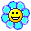battle programmers alliance
Would you like to react to this message? Create an account in a few clicks or log in to continue.

battle programmers alliance

# vb.net check if two rectangles overlap

function code :

Code:

`Public Shared Function overlappingRectangles(ByVal R1 As Rectangle, ByVal R2 As Rectangle) As Boolean            If R1.X > (R2.X + R2.Width) Or (R1.X + R1.Width) < R2.X Then                Return False            End If            If R1.Y > (R2.Y + R2.Height) Or (R1.Y + R1.Height) < R2.Y Then                Return False            End If            Return True        End Function`

summon code

Code:

`Dim R1 As New Rectangle(0, 0, 10, 10)        Dim R2 As New Rectangle(-8, -5, 300, 200)        Dim Bool2 As Boolean = Aeye.overlappingRectangles(R1, R2)        MessageBox.Show(CStr(Bool2))`

the walkthrough assumes you put the function in the A.Eye named class_________________
MB over and outDim R1 As New Rectangle(0, 0, 10, 10) 'x,y,width,height

_________________
MB over and outprivacy_tip Permissions in this forum:
You cannot reply to topics in this forum